# 深入浅出贝塞尔曲线

## 贝塞尔曲线的定义及推导过程

贝塞尔曲线于1962年，由法国工程师皮埃尔·贝兹（Pierre Bézier）所广泛发表，他运用贝塞尔曲线来为汽车的主体进行设计。贝塞尔曲线最初由保尔·德·卡斯特里奥于1959年运用德卡斯特里奥算法开发，以稳定数值的方法求出贝塞尔曲线。贝塞尔曲线由ｎ个控制点对应着ｎ－１阶的贝塞尔曲线，并且可以通过递归的方式来绘制。

下面先给出n阶贝塞尔曲线的公式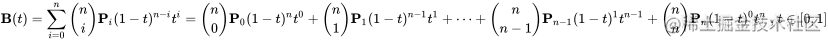### 一阶贝塞尔曲线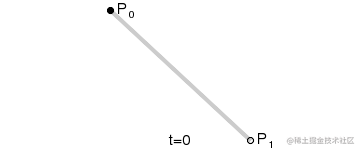设定图中运动的点为$P_t$$t$为运动时间，$t∈(0,1$)，可得如下公式

$P_t=P_0+\left(P_1-P_0\right)t=\left(1-t\right)P_0+P_1t \tag {1}$

### 二阶贝塞尔曲线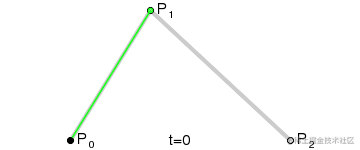二阶贝塞尔曲线由$P_0$$P_1$$P_2$三个点来确定，其中$P_0$为起点，$P_2$为终点，$P_1$为控制点，曲线方程为：

$P_t= \left(1-t\right)^2P_0+2t\left(1-t\right)P_1+t^2P_2 \quad\quad t\in\left(0,1\right)$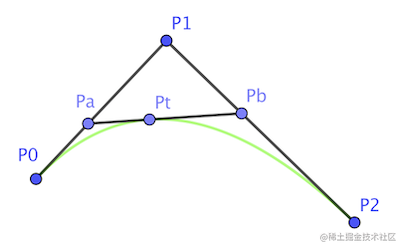1. 已知三个点$P_0$$P_1$$P_2$，连接线段$P_0P_1$$P_1P_2$
2. $P_a$$P_0P_1$上，随时间t从$P_0$运动到$P_1$，使得$P_0P_a/P_0P_1=t$
3. $P_b$$P_1P_2$上，随时间t从$P_1$运动到$P_2$，使得$P_1P_b/P_1P_2=t$
4. 连接线段$P_aP_b$
5. $P_t$$P_aP_b$上，随时间t从$P_a$运动到$P_b$，使得$P_aP_t/P_aP_b=t$
6. $t$从0变化到1的过程中，所有$P_t$点就组成了二阶贝塞尔曲线。

由公式（1）可得

$P_a=P_0+\left(P_1-P_0\right)t=\left(1-t\right)P_0+P_1t \tag {2}$
$P_b=P_1+\left(P_2-P_1\right)t=\left(1-t\right)P_1+P_2t \tag {3}$
$P_t=P_a+\left(P_b-P_a\right)t=\left(1-t\right)P_a+P_bt \tag {4}$

将公式（2）和公式（3）代入公式（4）可得

\begin{aligned} P_t&=P_a+\left(P_b-P_a\right)t=\left(1-t\right)P_a+P_bt \\ &=\left(1-t\right) \left[ \left(1-t\right)P_0+P_1t \right]+t \left[ \left( 1-t\right)P_1+P2t\right]\\ &=\left(1-t\right)^2P_0+2t\left(1-t\right)P_1+t^2P_2 \end{aligned}

### 三阶贝塞尔曲线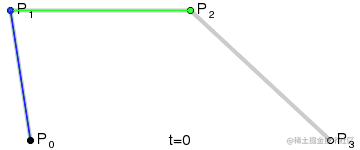三阶贝塞尔曲线由$P_0$$P_1$$P_2$$P_3$四个点来确定，其中$P_0$为起点，$P_3$为终点，$P_1$$P_2$为控制点，曲线方程为：

$P_t= \left(1-t\right)^3P_0+3t\left(1-t\right)^2P_1+3t^2\left(1-t\right)P_2+t^3P_3 \quad\quad t\in\left(0,1\right)$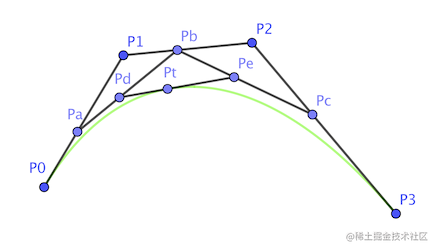1. 已知三个点$P_0$$P_1$$P_2$$P_3$，连接线段$P_0P_1$$P_1P_2$以及$P_2P_3$
2. $P_a$$P_0P_1$上，随时间t从$P_0$运动到$P_1$，使得$P_0P_a/P_0P_1=t$
3. $P_b$$P_1P_2$上，随时间t从$P_1$运动到$P_2$，使得$P_1P_b/P_1P_2=t$
4. $P_c$$P_2P_3$上，随时间t从$P_2$运动到$P_3$，使得$P_2P_c/P_2P_3=t$
5. 连接线段$P_aP_b$$P_bP_c$
6. $P_d$$P_aP_b$上，随时间t从$P_a$运动到$P_b$，使得$P_aP_d/P_aP_b=t$
7. $P_e$$P_bP_c$上，随时间t从$P_b$运动到$P_c$，使得$P_bP_e/P_bP_c=t$
8. $P_t$$P_dP_e$上，随时间t从$P_d$运动到$P_e$，使得$P_dP_t/P_dP_e=t$
9. $t$从0变化到1的过程中，所有$P_t$点就组成了三阶贝塞尔曲线。

由之前的公式可得：

\begin{aligned} P_a&=P_0+\left(P_1-P_0\right)t=\left(1-t\right)P_0+P_1t \\ P_b&=P_1+\left(P_2-P_1\right)t=\left(1-t\right)P_1+P_2t \\ P_c&=P_2+\left(P_3-P_2\right)t=\left(1-t\right)P_2+P_3t \\ P_d&=P_a+\left(P_b-P_a\right)t=\left(1-t\right)P_a+P_bt \\ P_e&=P_b+\left(P_c-P_b\right)t=\left(1-t\right)P_b+P_ct \\ P_t&=P_d+\left(P_e-P_d\right)t=\left(1-t\right)P_d+P_et \\ \end{aligned}

将上述公式带入$P_t$可得

\begin{aligned} P_t&= \left(1-t\right)P_d+P_et \\ &=\left(1-t\right)\left[\left(1-t\right)P_a+P_bt \right]+t\left[ \left(1-t\right)P_b+P_ct \right]\\ &=\left(1-t\right)^2P_a+2t\left(1-t\right)P_b+t^2P_c\\ &=\left(1-t\right)^2\left[ \left(1-t\right)P_0+P_1t \right]+2t\left(1-t\right)\left[ \left(1-t\right)P_1+P_2t \right]+t^2\left[ \left(1-t\right)P_2+P_3t \right]\\ &=\left(1-t\right)^3P_0+t\left(1-t\right)^2P_1+2t\left(1-t\right)^2P_1+2t^2\left(1-t\right)P_2+t^2\left(1-t\right)P_2+t^3P_3\\ &=\left(1-t\right)^3P_0+3t\left(1-t\right)^2P_1+3t^2\left(1-t\right)P_2+t^3P_3 \quad\quad t\in\left(0,1\right) \end{aligned}

### 递归性质

仔细观察上述的构造过程，经过第5步变化之后，三阶贝塞尔曲线的求解变成了对以$P_a$为起点，$P_c$为终点，$P_b$为控制点的二阶贝塞尔曲线方程的求解。

首先，有四个控制点；

我们发现，实际上是每轮都是 n 个点，形成 n-1 条线段，每个线段上有一个点在运动，那么就只关注这 n-1 个点，循环往复。最终只剩一个点时，它的轨迹便是结果。

通过对上面贝塞尔曲线的定义及推导过程的阅读，我们对贝塞尔曲线是什么以及如何获得相应阶数的曲线公式有了初步的认识，那么在日常开发中我们如何定义并使用贝塞尔曲线呢？UIBezierPathUIKit中的一个关于图形绘制的类，是对CGPathRef的封装，可以方便的让我们画出 矩形、椭圆或者直线和曲线的组合形状。下面我们简单介绍一下UIBezierPath的常用api。

### UIBezierPath

UIBezierPath用于定义一个直线和曲线组合而成的路径，并且可以在自定义视图中渲染该路径。

#### 常用api

//创建并返回一个新的bezierPath对象
+ (instancetype)bezierPath;

//通过一个矩形rect创建并返回一个矩形bezierPath对象
+ (instancetype)bezierPathWithRect:(CGRect)rect;

//通过一个矩形rect创建并返回一个与该矩形内接的椭圆bezierPath对象
+ (instancetype)bezierPathWithOvalInRect:(CGRect)rect;

//通过一个已存在的路径，返回一个该路径的反转路径
- (UIBezierPath *)bezierPathByReversingPath;

//移动path的currentPoint到指定的位置
- (void)moveToPoint:(CGPoint)point;

//在路径中添加一条直线，从currentPoint开始到指定位置

//在路径中添加一条二阶贝塞尔曲线，以endPoint为终点，controlPoint为控制点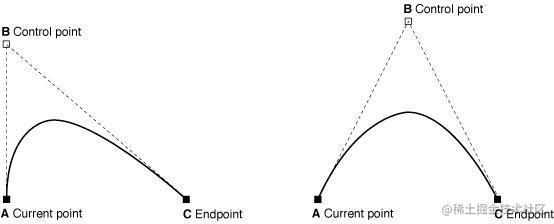//在路径中添加一条三阶贝塞尔曲线，以endPoint为终点，controlPoint1和controlPoint2为两个控制点，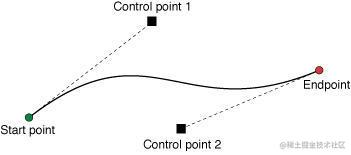//闭合路径，从currentPoint到路径起点添加一条直线
- (void)closePath;

//移除路径上所有点，即删除所有子路径
- (void)removeAllPoints;

//在路径中添加另一条路径
- (void)appendPath:(UIBezierPath *)bezierPath;

//获取路径的不可变CGPathRef对象
@property(nonatomic) CGPathRef CGPath;

//路径绘制过程中的当前点，即下次绘制的起点，如果路径为空，该属性值为CGPointZero

//路径线宽，默认值1.0
@property(nonatomic) CGFloat lineWidth;

//路径曲线起点和终点样式，只对开放路径起作用，对闭合路径无效，默认值为 kCGLineCapButt
@property(nonatomic) CGLineCap lineCapStyle;

typedef CF_ENUM(int32_t, CGLineCap) {
kCGLineCapButt,//方形末端，结束位置在精确位置
kCGLineCapRound,//圆形末端，结束位置超过精确位置半个线宽
kCGLineCapSquare//方形末端，结束位置超过精确位置半个线宽
};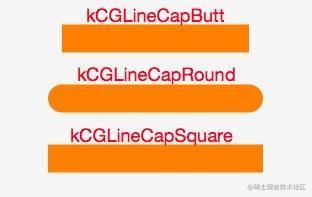//路径线段的连接点样式，默认值为 kCGLineJoinMiter
@property(nonatomic) CGLineJoin lineJoinStyle;

typedef CF_ENUM(int32_t, CGLineJoin) {
kCGLineJoinMiter,//尖角
kCGLineJoinRound,//圆角
kCGLineJoinBevel//切角
};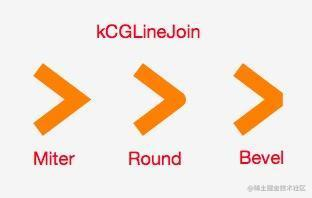//使用指定的仿射变换矩阵变换路径上的所有点
- (void)applyTransform:(CGAffineTransform)transform;

//填充路径
- (void)fill;

//绘制路径
- (void)stroke;

### 定义了path之后如何绘制到屏幕上？

1. 在UIView的- (void)drawRect:(CGRect)rect方法里面绘制图形
2. 使用CAShapeLayer

CAShapeLayerdrawRect比较：

• CAShapeLayer：属于CoreAnimation框架，通过GPU来渲染图形，节省性能，高效使用内存。
• drawRect：属于Core Graphics框架，占用大量CPU，耗费性能。

### CAShapeLayer

CAShapeLayer继承自CALayerCAShapeLayer属于CoreAnimation框架，通过GPU来渲染图形，节省性能。动画渲染直接提交给手机GPU，高效使用内存。 每个CAShapeLayer对象都代表着将要被渲染到屏幕上的一个任意的形状（shape）。具体的形状由其path（类型为CGPathRef）属性指定。 普通的CALayer是矩形，需要frame属性。CAShapeLayer初始化时也需要指定frame值，但它本身没有形状，它的形状来源于其属性path 。CAShapeLayer中shape需要形状才能生效。UIBezierPath可以为其提供绘制形状的path。

### 常用api

//图层渲染的路径 Animatable，对path进行动画时，要注意保证前后两个path拥有相同数量的控制点
@property(nullable) CGPathRef path;

//图层路径颜色
@property(nullable) CGColorRef strokeColor;

//路径渲染的起止相对位置，取值在[0,1]之间，可动画
@property CGFloat strokeStart;
@property CGFloat strokeEnd;

//开始绘制位置在虚线长度中的位置
@property CGFloat lineDashPhase;

//虚线的规格，数组定义了实线和空格的宽度
@property(nullable, copy) NSArray<NSNumber *> *lineDashPattern;

//线宽，意义同UIBezierPath
@property CGFloat lineWidth;

//起点和终点样式，意义同UIBezierPath
@property(copy) CAShapeLayerLineCap lineCap;

//连接点样式，意义同UIBezierPath
@property(copy) CAShapeLayerLineJoin lineJoin;

## 举个例子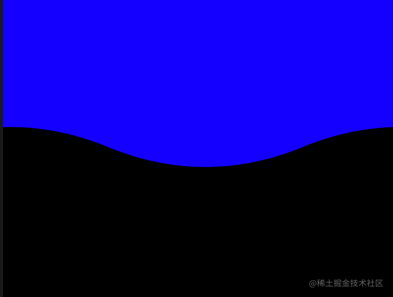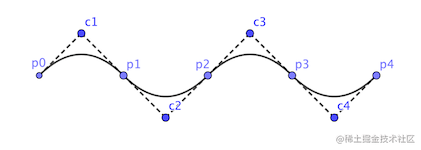下面我们将用二阶和三阶贝塞尔曲线实现上面的波浪动图效果，如上示意图所示，将波浪曲线进行分解，通过绘制两个相同的完整的“正弦波”，然后不停的将曲线向左侧移动和复位，来达到波浪起伏的效果。

### 二阶实现

// p0p1，p1p2，p2p3，p3p4为4条二阶曲线，c1，c2，c3，c4为其相应二阶贝塞尔曲线的控制点

- (void)p_creatWavePath {
CGFloat waterHeight = 300.f;
CGFloat waveWidth = self.view.frame.size.width / 2.f;
CGFloat waveControlHeight = 50.f;

UIBezierPath *wavePath = [UIBezierPath bezierPath];
[wavePath moveToPoint:CGPointMake(0 - self.waveOffsetX, 0)];

// 计算起点和控制点
CGFloat waveHeight = 20.f;
CGPoint p0 = CGPointMake(0 - self.waveOffsetX, waterHeight);
CGPoint p1 = CGPointMake(waveWidth - self.waveOffsetX, waterHeight);
CGPoint p2 = CGPointMake(waveWidth * 2 - self.waveOffsetX, waterHeight);
CGPoint p3 = CGPointMake(waveWidth * 3 - self.waveOffsetX, waterHeight);
CGPoint p4 = CGPointMake(waveWidth * 4 - self.waveOffsetX, waterHeight);
CGPoint c1 = CGPointMake(waveWidth * 1 / 2 - self.waveOffsetX, waterHeight - waveControlHeight);
CGPoint c2 = CGPointMake(waveWidth * 3 / 2 - self.waveOffsetX, waterHeight + waveControlHeight);
CGPoint c3 = CGPointMake(waveWidth * 5 / 2 - self.waveOffsetX, waterHeight - waveControlHeight);
CGPoint c4 = CGPointMake(waveWidth * 7 / 2 - self.waveOffsetX, waterHeight + waveControlHeight);

[wavePath addLineToPoint:CGPointMake(waveWidth * 4 - self.waveOffsetX, 0)];
[wavePath closePath];
self.waveLayer.path = wavePath.CGPath;
// 累加曲线的向左的偏移量
self.waveOffsetX = (self.waveOffsetX + self.waveStepX) % (NSInteger)(self.view.frame.size.width);
}

## 三阶实现

// p0p1p2，p2p3p4为两条三阶阶曲线，c1和c2，c3和c4为其相应三阶贝塞尔曲线的控制点

- (void)p_creatWavePath {
CGFloat waterHeight = 300.f;
CGFloat waveWidth = self.view.frame.size.width / 2.f;
CGFloat waveControlHeight = 50.f;

UIBezierPath *wavePath = [UIBezierPath bezierPath];
[wavePath moveToPoint:CGPointMake(0 - self.waveOffsetX, 0)];

// 计算起点和控制点
CGPoint p2 = CGPointMake(waveWidth * 2 - self.waveOffsetX, waterHeight);
CGPoint p4 = CGPointMake(waveWidth * 4 - self.waveOffsetX, waterHeight);
CGPoint c1 = CGPointMake(waveWidth  * 1 / 2 - self.waveOffsetX, waterHeight - waveControlHeight);
CGPoint c2 = CGPointMake(waveWidth * 3 / 2 - self.waveOffsetX, waterHeight + waveControlHeight);
CGPoint c3 = CGPointMake(waveWidth * 5 / 2 - self.waveOffsetX, waterHeight - waveControlHeight);
CGPoint c4 = CGPointMake(waveWidth * 7 / 2 - self.waveOffsetX, waterHeight + waveControlHeight);

[wavePath addLineToPoint:CGPointMake(waveWidth * 4 - self.waveOffsetX, 0)];
[wavePath closePath];
self.waveLayer.path = wavePath.CGPath;
self.waveOffsetX = (self.waveOffsetX + self.waveStepX) % (NSInteger)(self.view.frame.size.width);
}

## 反推控制点

由之前的介绍可知，要绘制一条贝塞尔曲线，除了起点和终点外，还必须要知道相应数量的控制点。但在日常开发中我们并不是总能知道控制点，取而代之的是一些曲线经过的点，这个时候要怎么办呢？下面以三阶曲线为例，推导控制点的计算过程

$P_t= \left(1-t\right)^3P_0+3t\left(1-t\right)^2P_1+3t^2\left(1-t\right)P_2+t^3P_3 \quad\quad t\in\left(0,1\right)$

移动方程式可得：

$3t\left(1-t\right)^2P_1+3t^2\left(1-t\right)P_2 = P_t - \left(1-t\right)^3P_0 - t^3P_3 \quad\quad$

假设已知三阶贝塞尔曲线的起点$P_0$，终点$P_3$，t=1/4时曲线上的点$P_a$，t=3/4时曲线上的点$P_b$

\begin{aligned} 27/64*P_1 + 9/64*P_2 &= P_a - 27/64*P_0 - 1/64*P_3 \\ 27P_1+9P_2 &= 64P_a-27P_0-P_3 \\ \end{aligned}

设$P_c=64P_a-27P_0-P_3$，由于$P_a$$P_0$$P_3$已知，$P_c$也可以通过计算得出；即

$27P_1+9P_2=P_c \tag {5}$

同理将t=3/4时的点$P_b$带入公式可得：

\begin{aligned} 9/64*P_1 + 27/64*P_2 &= P_b - 1/64*P_0 - 27/64*P_3 \\ 9P_1+27P_2 &= 64P_b-P_0-27P_3 \\ \end{aligned}

设$P_d=64P_b-P_0-27P_3$， 由于$P_b$$P_0$$P_3$已知，$P_d$也可以通过计算得出；即

$9P_1+27P_2 = P_d \tag {6}$

将公式(5)和公式(6)代入化简可得：

$P_1=\left(3P_a-P_b\right)/72 \\ P_2=\left(3P_b-P_a\right)/72$

下面是上述例子中控制点求解的函数实现

// 三阶曲线求控制点
// p0：起点，p3：终点，pa：t=t1时曲线上的点，pb：t=t2时曲线上的点
- (NSArray<NSValue *> *)p_calculateControlPointsWithPoint0:(CGPoint)p0 pointA:(CGPoint)pa t1:(CGFloat)t1 pointB:(CGPoint)pb t2:(CGFloat)t2 point3:(CGPoint)p3 {
// ax + by = c
// dx + ey = f
// x = (b * f - c * e) / (b * d - a * e)
// y = (c * d - a * f) / (b * d - a * e)

CGFloat fa = 3 * t1 * pow((1 - t1), 2);
CGFloat fb = 3 * (1 - t1) * pow(t1, 2);
CGFloat fd = 3 * t2 * pow((1 - t2), 2);
CGFloat fe = 3 * (1 - t2) * pow(t2, 2);

CGFloat fcx = pa.x - pow((1 - t1), 3) * p0.x - pow(t1, 3) * p3.x;
CGFloat fcy = pa.y - pow((1 - t1), 3) * p0.y - pow(t1, 3) * p3.y;
CGFloat ffx = pb.x - pow((1 - t2), 3) * p0.x - pow(t2, 3) * p3.x;
CGFloat ffy = pb.y - pow((1 - t2), 3) * p0.y - pow(t2, 3) * p3.y;

CGPoint p1 = CGPointZero;
CGPoint p2 = CGPointZero;
p1.x = (fb * fcx - ffx * fe) / (fb * fd - fa * fe);
p1.y = (fb * fcy - ffy * fe) / (fb * fd - fa * fe);
p2.x = (fd * fcx - ffx * fa) / (fb * fd - fa * fe);
p2.y = (fd * fcy - ffy * fa) / (fb * fd - fa * fe);

return @[[NSValue valueWithCGPoint:p1], [NSValue valueWithCGPoint:p2]];
}

hi, 我是快手电商的键盘破风手